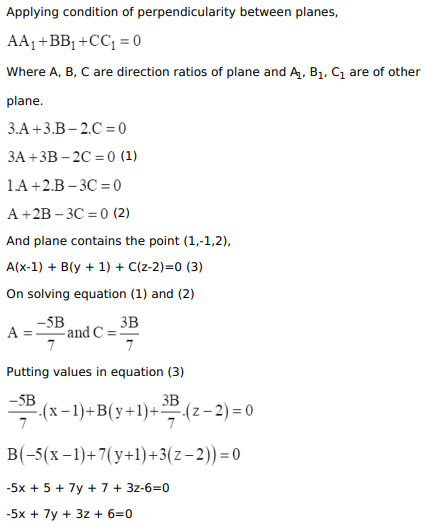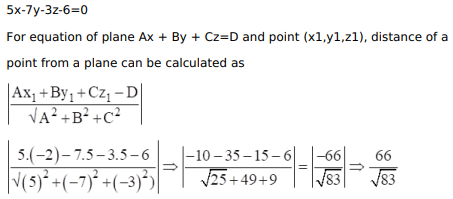# Find the equation of the plane that contains the pointQuestion:

Find the equation of the plane that contains the point $A(1,-1,2)$ and is perpendicular to both the planes $3 x+3 y-2 z=5$ and $x+2 y-3 z=$ 8. Hence, find the distance of the point $P(-2,5,5)$ from the plane obtained above.

Solution: